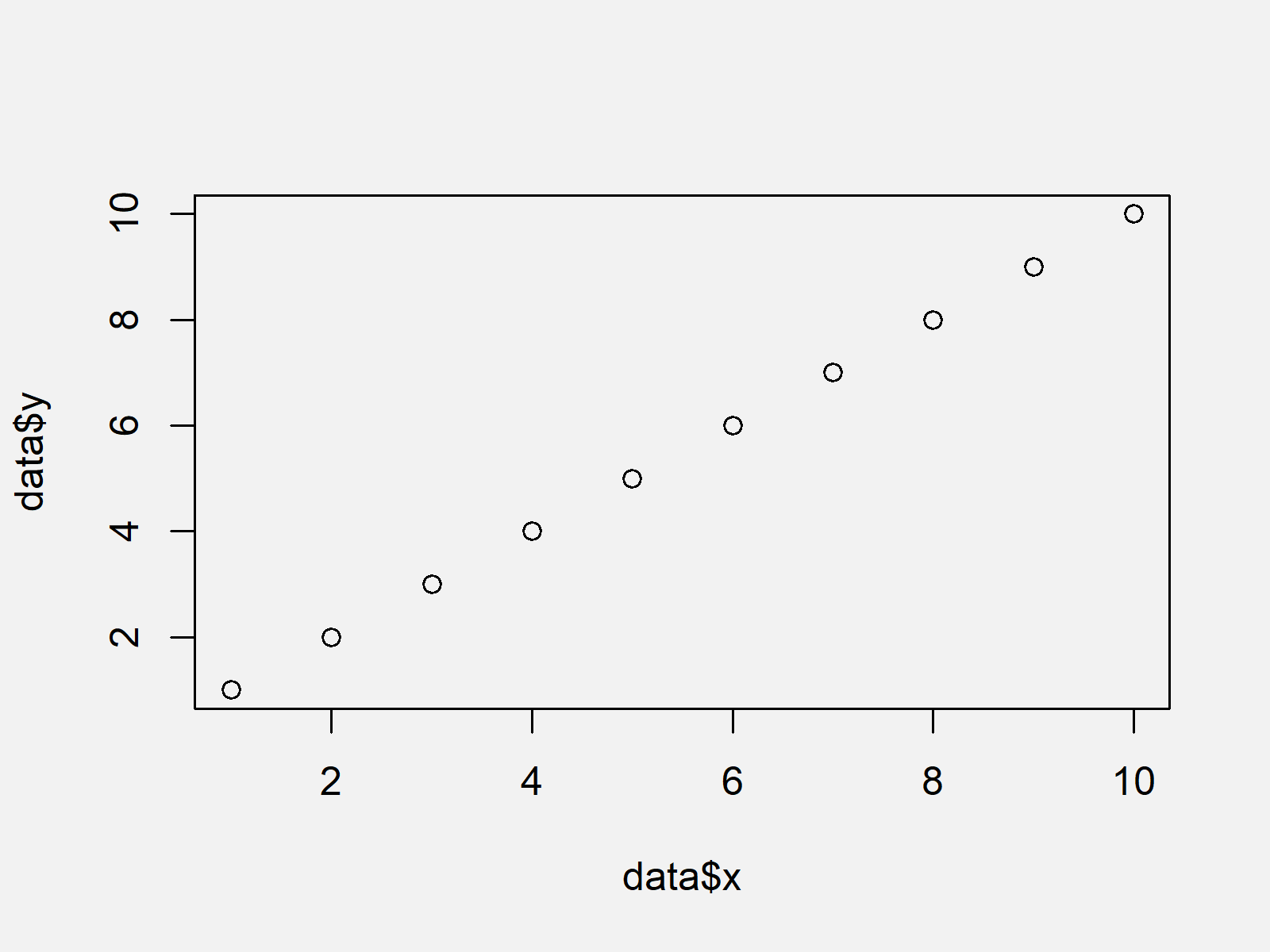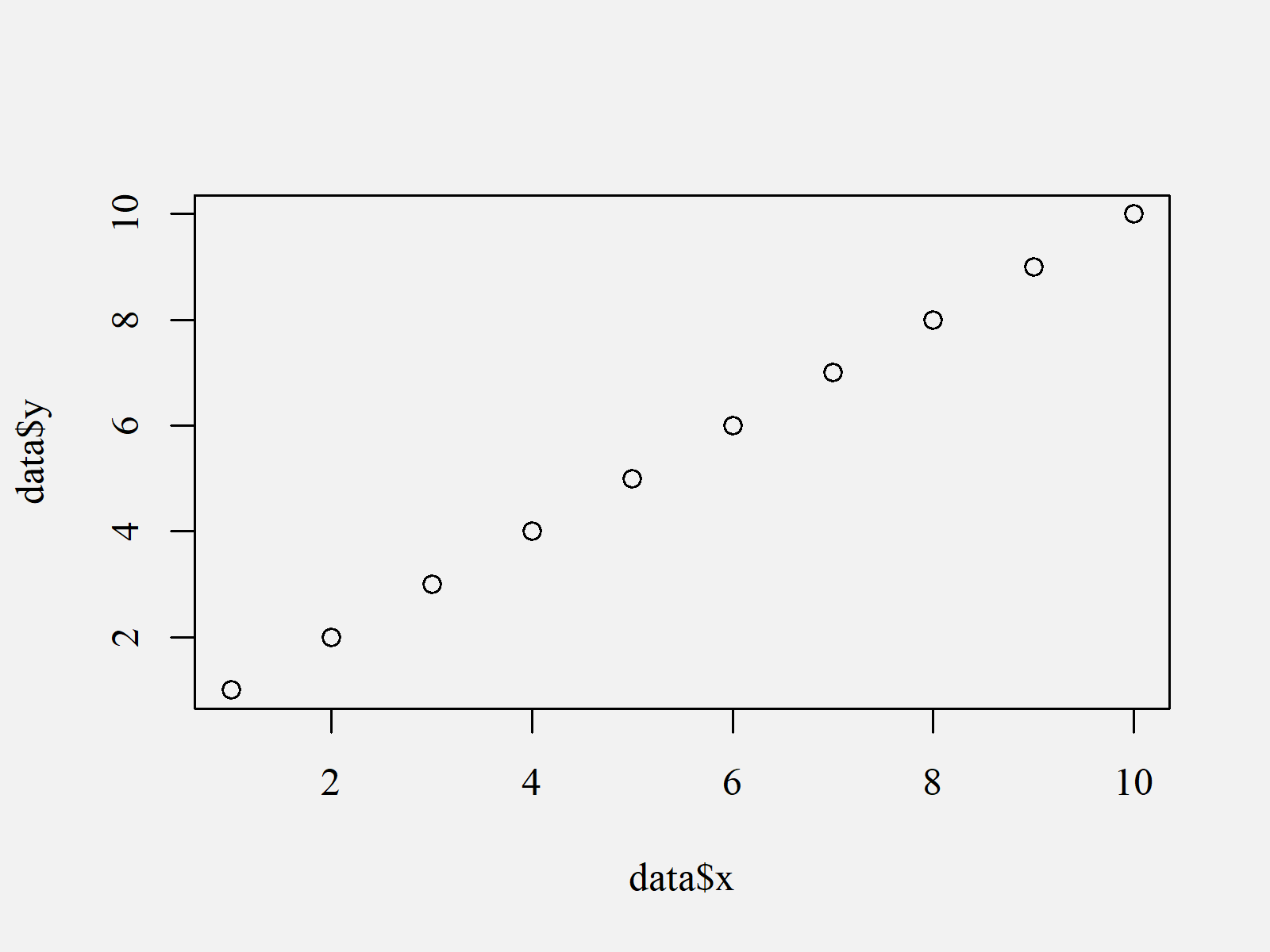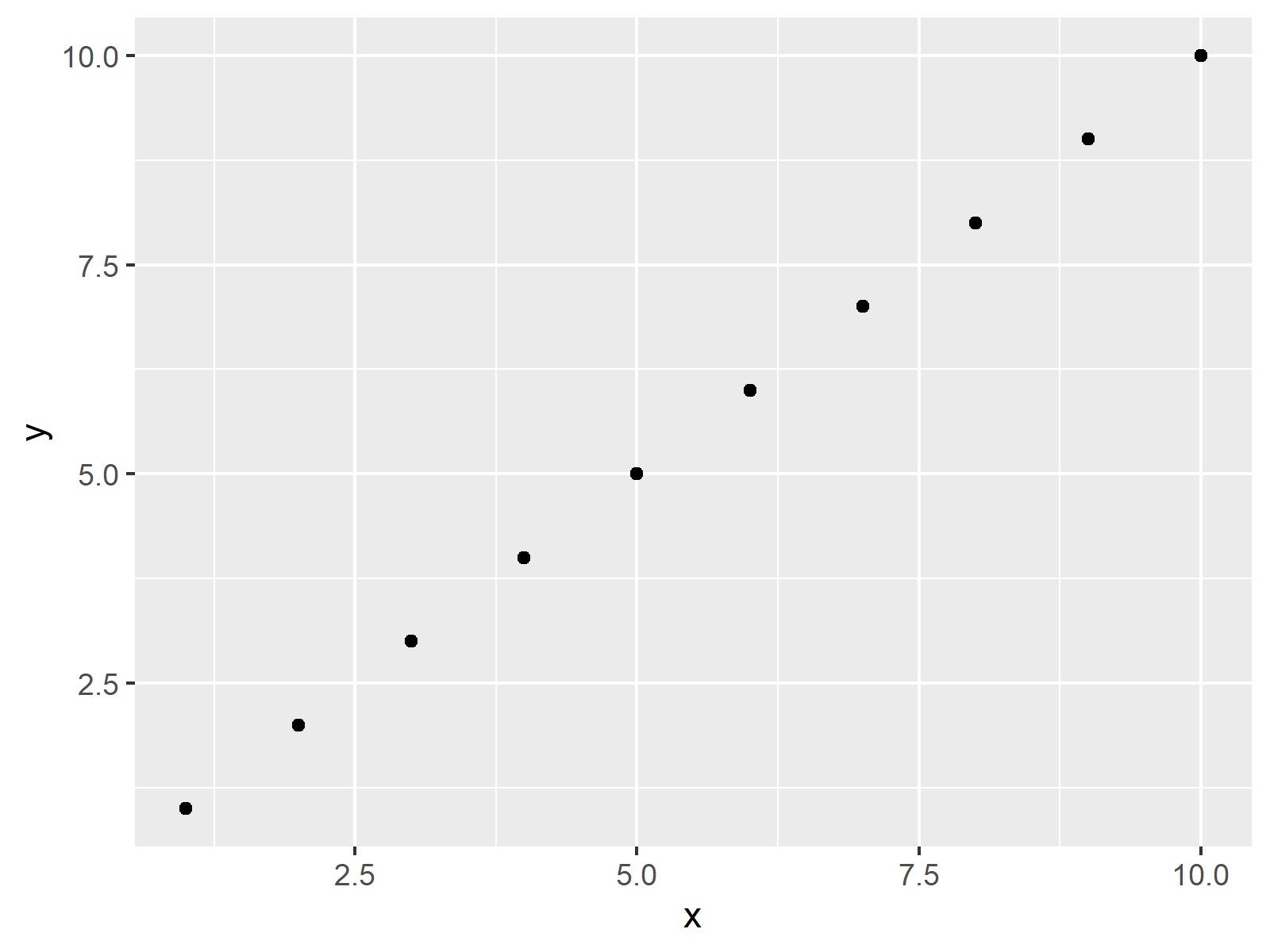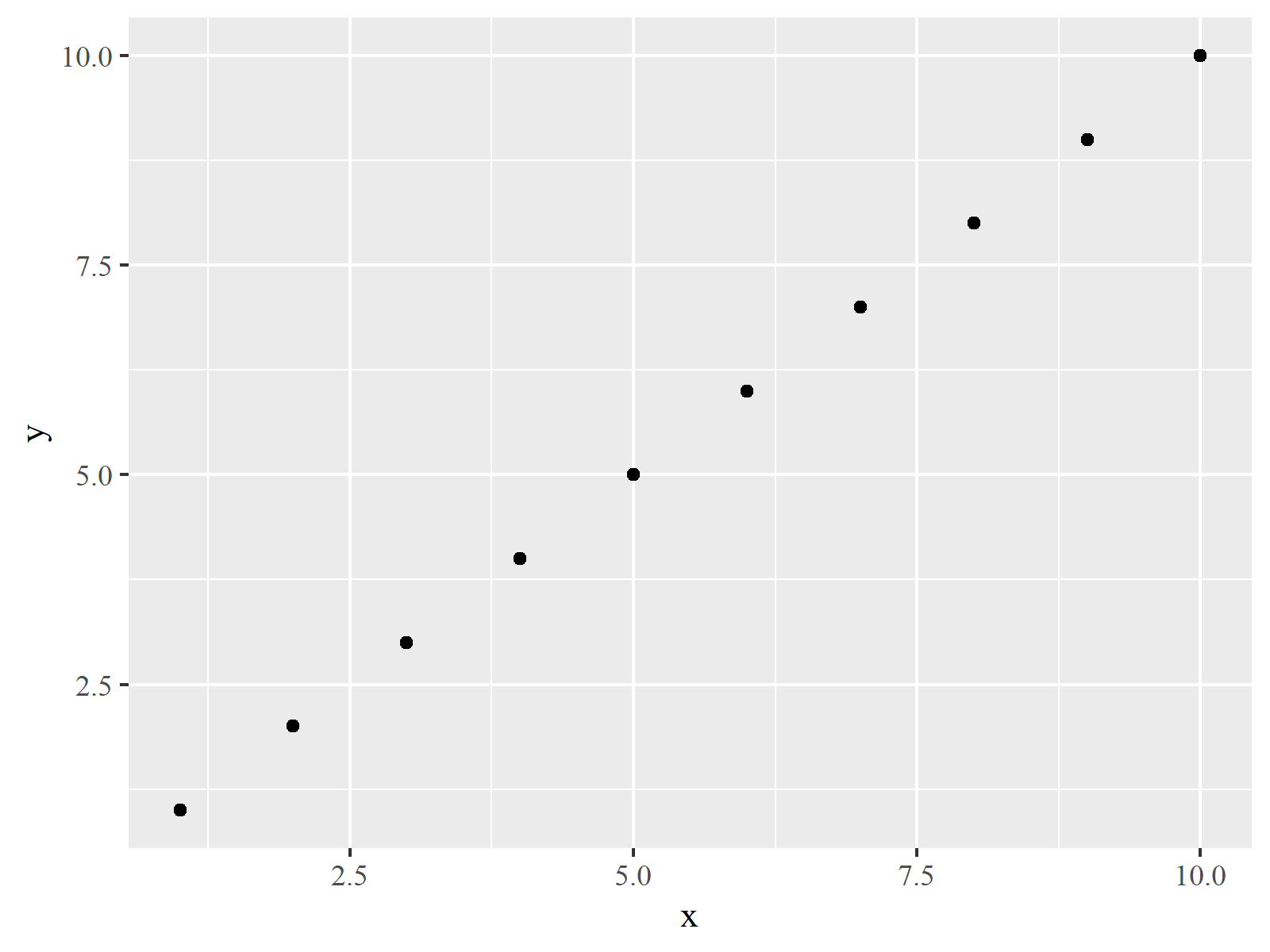# Change Font of Plot in R (3 Examples)

In this tutorial, I’ll illustrate how to select fonts of plots manually in R.

The article will contain three examples for the modification of font families in plots. More precisely, the content looks as follows:

You’re here for the answer, so let’s get straight to the examples.

## Creation of Example Data

As a first step, we need to construct some example data:

```data <- data.frame(x = 1:10,                      # Create example data
y = 1:10)
data                                              # Print example data
#     x  y
# 1   1  1
# 2   2  2
# 3   3  3
# 4   4  4
# 5   5  5
# 6   6  6
# 7   7  7
# 8   8  8
# 9   9  9
# 10 10 10```

As you can see based on the previous output of the RStudio console, our example data has ten rows and two columns. Both variables of our data frame contain a numeric range from 1 to 10.

## Example 1: Changing Font of Base R Plot

In Example 1, I’ll explain how to change the font family of a Base R graph.

Let’s first create a plot of our data with the default font specifications:

```plot(data\$x,                                      # Plot with default font
data\$y)```As shown in Figure 1, we created a default scatterplot with the previous R syntax.

If we want to change the fonts of all elements of our plot, we can use the windowsFonts function as shown below:

```windowsFonts(A = windowsFont("Times New Roman"))  # Specify font
plot(data\$x,                                      # Plot with manually specified font
data\$y,
family = "A")```As visualized in Figure 2, the previously shown R programming syntax created a Base R graph with the font Times New Roman.

## Example 2: Changing Font of ggplot2 Plot

In Example 2, I’ll show how to adjust the text font in a ggplot2 plot.

If we want to use the functions of the ggplot2 package, we first need to install and load ggplot2:

```install.packages("ggplot2")                       # Install & load ggplot2 package
library("ggplot2")```

Now, we can draw a ggplot2 scatterplot with default text specifications as shown below:

```ggp <- ggplot(data, aes(x, y)) +                  # ggplot2 plot with default font
geom_point()
ggp                                               # Draw ggplot2 plot```As revealed in Figure 3, the previous R code created a ggplot2 scatterplot with the typical font style.

As in Example 1, we can now use the windowsFonts command to change the font of our ggplot2 plot:

```windowsFonts(A = windowsFont("Times New Roman"))  # Specify font
ggp +                                             # ggplot2 plot with manually specified font
theme(text = element_text(family = "A"))```The output of the previous R code is shown in Figure 4: A ggplot2 graph with user-defined font.

## Example 3: Changing Font when Exporting Plot Using pdf() Function

In case you want to export your plot to an external file (such as PDF, JPEG, JPG etc.), you may modify the font family during the exportation process.

In this example, I’ll illustrate how to write a PDF file with manually specified font.

Have a look at the following R syntax:

```pdf("ggp.pdf", family = "Times")                  # Apply pdf function
ggp
dev.off()```

After running the previous R code, you should find a PDF file in your working directory that looks exactly as Figure 4 (i.e. a ggplot2 scatterplot with Times New Roman font).

## Video & Further Resources

If you need more info on the R programming syntax of this article, you may want to have a look at the following video of my YouTube channel. I’m explaining the content of this tutorial in the video:

Note that we have created only scatterplots in the examples of this tutorial. However, the same logic could be applied to other types of plots such as boxplots, barcharts, density plots, line plots, histograms, and so on.

Furthermore, it would also be possible to use other font types such as Calibri, Helvetica, Futura, Garamond, or Arial.

However, in addition to the video you may have a look at the related tutorials of this website:

This tutorial showed how to adjust the font family in Base R and ggplot2 graphics in R programming. Let me know in the comments section, if you have additional questions.

Subscribe to the Statistics Globe Newsletter

•margo
April 24, 2021 9:53 pm

Your site is so helpful, I use it a lot. Thank you for your hard work! I finally removed annoying back ground and lines from plot generated by ggplot. Thank you very much.

•April 26, 2021 6:18 am

Hi Margo,

Thanks a lot for the great feedback, glad to hear that it helped! 🙂

Regards

Joachim

•Jenn
August 2, 2023 4:00 pm

Thank you for the tutorials!

THis is helpful, but I’m still having issues… I have used the exact code above (for Base) and, while I get no errors in the code, the font on the figure output does not change. I’m using RStudio, if that helps.

•August 8, 2023 9:27 am